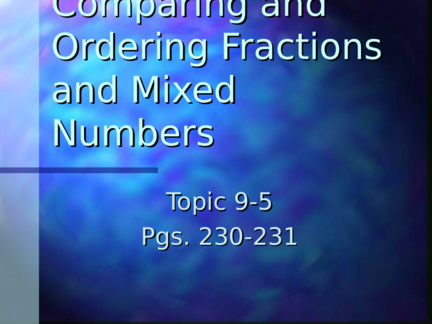# Comparing and Ordering Fractions and Mixed NumbersContributed by:This ppt explains, how to compare fractions and order them by using examples. A fraction represents a part of a whole or, more generally, any number of equal parts. A fraction describes how many parts of a certain size there are, for example, one-half, eight-fifths, three-quarters.
1. Comparing and
Ordering Fractions
and Mixed
Topic 9-5
Pgs. 230-231
2. How can you compare
 Problem: Shawna and Tom walked
two different paths in Trout park.
Shawna walked 5/6 mile. Tom
walked ¾ mile. Which is greater,
5/6 or ¾?
3. Which is greater 5/6 or ¾?
 One Way  Multiply your
fractions by the
To compare fractions,
number it takes to
find a common get 12 as a
denominator by denominator
writing the multiples  5 x 2 = 10
of each denominator 6 2 12
4: 4, 8, 12, 16, 20… 3 x 3 = 9
6: 6, 12, 16, 18, 24… 4 3 12
Use 12 as the common 10/12 > 9/12 so,
denominator 5/6 > 3/4
4. Which is greater 5/6 or ¾?
 Another Way: Multiply your fractions
You can multiply the by the number it
takes to get 24 as
denominators to the denominator
find a common
5 x 4 = 20
denominator.
6 4 24
Compare ¾ and 5/6
3 x 6 = 18
Multiply denominators:
4 x 6 24
4 x 6= 24 20/24 > 18/24 so
Use 24 as the common 5/6 > 3/4
denominator
5. How can you order
fractions and mixed
 Write 2 5/12, 11/12, 3 1/6, 2 1/3 in order from least to
greatest
 You know that 11/12 < 1 and all the mixed numbers are
greater than 1. So 11/12 is the least number
 When comparing mixed numbers, look at the whole number
parts. Since 3>2, you know that 3 1/6 is greater than both 2
1/3 and 2 5/12
 Next, compare 2 1/3 and 2 5/12. Since the whole numbers
are the same, compare the fractions.
 Compare 1/3 and 5/12. Change 1/3 to 4/12
 4/12 < 5/12 so 2 1/3 < 2 5/12
 From greatest to least, the numbers are 3 1/6, 2 5/12, 2 1/3,
11/12
6. Let’s Try it Together
 Compare the  Order from least
following to greatest
fractions 1) 2 1 9
1. 3 4 3 , 4 , 10
5 5 Answer: ¼, 2/3, 9/10
<, >, or =? 2) 1 2 2 1 1 9
2. 1 2 3 10 4
Answers: 1 2/3, 1 9/10, 2 1/4
4 3
7. Independent Practice #7-
 Use your notes to determine the
 We will review the answers in 10
minutes
 Good Luck!
8. Common Factors
and Greatest
Common Factors
9-6
Pgs. 232- 233
9.  Ifa number is a factor of two
numbers, it is called a common
factor
 The greatest common factor (GCF)
of two numbers is the greatest
number that is a factor of both
numbers
10. How can you find the
greatest common factor of
20 and 30?
 Problem: A pet store has  One Way:
goldfish and angelfish To find the greatest common
that have to be put into factor of 20 and 30, you
the fewest number of can list all the factors of
glass containers. Each each and circle the
container must contain numbers they have in
common
the same number of fish,
20: 1, 2, 4, 5, 10, 20
and each must contain all
goldfish or all angelfish. 30: 1, 2, 3, 5, 6, 10, 15, 30
Of all the numbers they have
 Find the GCF of 20 and in the common what is the
30 largest number?
10 so the GCF of 20 and
30=10
11. How can you find the
greatest common factor of
20 and 30?
 Another way is to use prime factorization to find the
GCF
 Find GCF of 24 and 18 (pg. 232)
1. Find the prime factors of each number
2. List the prime factors of each number
24= 2 x 2 x 2 x 3
18= 2 x 3 x 3
3. Circle the prime factors that both numbers share which
are 2 and 3
4. Multiply the common factors: 2 x 3=6
So, the GCF of 18 and 24 is 6
12. Let’s Try it Together!
 Find the GCF of  Answers:
each pair of
numbers
1. 3
1) 9 and 12 2. 5
2) 20 and 45 3. 7
3) 7 and 28 4. 2
4) 18 and 32
5) If two numbers are
prime, what is their
GCF?
13. Independent Practice
 Complete #7-14 and we will review
them as a class
 Good Luck